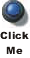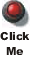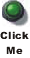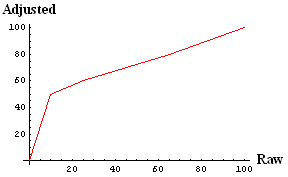# PHY138 - Mechanics Test

This document discusses the test of October 28, 2003. First I discuss the questions, the marking scheme, and some comments. Then I talk about the results of the test.

## The Questions, Answers and Marking Scheme

 You may access a pdf version of the Multiple Choice section, including the answer key, by clicking on the button to the right. File size is 247k.You may access a pdf version of the Problem by clicking on the button to the right. File size is 168k.You may access a pdf version of the answers and marking scheme for the Problem by clicking on the button to the right. File size is 154k.In general the test was too long, which surprised us. We apologise.

Some student comments say that the Problem was all about material from Chapter 10 - Rotational Motion. Those students are wrong! Part A is from Chapter 4 except for last question; using the Work - Kinetic Energy theorem from Chapter 6 is an easier way to solve this problem. Part B extends Part A with material from Chapter 8, except for the last question.

I also have some specific comments about three of the Multiple Choice Questions:

### Multiple-Choice Question 1

In order for the students to cross the river in the minimum time, the component of their total velocity vector pointing across the river has to be a maximum. The total velocity vector is the vector sum of the velocity of the boat relative to the water plus the the velocity of the water relative to the riverbank. Since the velocity of the water only has a component parallel to the riverbank, we can ignore it in answering the question. So we are left with the question of what orientation of the velocity of the boat has a maximum component pointing across the river.

It should be clear, then, that the answer is d: directly toward the opposite shore.

We were surprised and disappointed that only 35% of the class got this question correct.

### Multiple-Choice Question 2

This was the problem about the 10 N weight hanging from a spring scale in an elevator that is accelerating upwards, and you were asked about the reading of the scale.

22% of the class chose the only answer that was less than 10N. This was the second-most popular choice, after the correct answer. Students who chose this wrong answer were clearly not thinking about the actual situation: the answer obviously has to be greater than 10N.

### Multiple-Choice Question 6

This question is very similar to the text's Quick Quiz 10.5, which we spent a fair amount of time discussing in class. We will solve the test question using similar reasoning to that we used in doing the Quick Quiz: note that the solution does not use any formulae.

First, we realise that since the change of potential energy of all 4 objects is the same, their kinetic energies will be the same at the bottom. The rotational kinetic energy is just 1/2 I2.

Since the kinetic energy is the same for all 4 objects, then the one with the smallest moment of inertia will have the largest angular velocity. So we need to know which object has the smallest moment of inertia.

Now, the moment of inertia is just a weighted average of all the mass of the body, where the weighting is the square of the distance of each small mass from the axis of rotation.

In the Quick Quiz we realised that the solid sphere has a smaller moment of inertia than the hollow one, since it has a larger portion of its mass at smaller distances from the axis of rotation.

We can similarly reason that the solid cylinder has a smaller moment of inertia than the hollow one.

So we now have the answer to the Question down to a choice between the solid sphere and the solid cylinder.

Imagine a "slice" of the cylinder, a thin disc. Each similar slice of the cylinder has the same moment of inertia.

The same "slices" of the sphere do not all have the same moment of inertia: the ones closer to the top and bottom of the sphere have their mass distributed closer to the axis than the slices closer to the equator of the sphere.

So, finally, the solid sphere has the smallest moment of inertia and is the answer to the question.

The most popular answer, chosen by 42% of the class, was that the angular velocities of all four objects were the same. This is obviously wrong, and we are disappointed that nearly half the class thought it was correct. The next most popular answer, chosen by only 21% of the class, was the correct one. We expected this question to be the hardest one in the Multiple Choice section, and it was. We did not expect it to be this hard, however.

## Test Results

Recall that the Quiz was too easy. The Test was too hard. We will try to make subsequent Tests and the Final Exam more like the temperature of Baby Bear's porridge: just right.

1033 students wrote the test. Here are some statistics on the "raw" results:

Statistic Value
Mean
42.7
Standard Deviation
21.5
Median
42.5
MInimum
0
Maximum
100

I think that this is a good class, although we are all disappointed in the results. We have rescaled the marks using the following "breakpoints:"

Raw Mark Scaled To:
0
0
10
50
25
60
45
70
65
80
100
100This leads to the following mark distributions:

What Value
Mean
67.5
Standard Deviation
14.7
Median
69
A's
18%
B's
29%
C's
30%
D's
16%
E's and F's
7%These adjusted marks are the ones that are stored on STORM and will be used in calculating your final mark in the course without further adjustments.

Recall that only the best 3 of the 4 tests count towards your final mark in the course. Thus if you did not do as well as you could have on this test, you will still have lots of opportunities to demonstrate your understanding of the content of PHY138.

This document was written by David M. Harrison on November 4, 2003